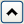Creates a list of values beginning with the from value and ending with the to value. The number of steps specifies the number of values in the list between and including the from and to values. Each value determined by the first three arguments of the count function occurs consecutively the number of times that you specify with the times argument. When the to value is reached, Count starts over at the from value.
Also, you can add the times argument with the insert buttonon the keyboard. This argument is one by default, but repeats the count process as many times as you specify, as illustrated by the Count4 column in the data table in Example of the Count Function. To add any argument to the Count function, highlight the argument preceding the one that you want to enter. Either type a comma or use the insert buttonon the Formula Editor keypad.
 • Count (1, 9, 2) gives Count 1
 • Count (1, 9, 3) gives Count 2
 • Count (1, 9, 9) gives Count 3
 • Count (1, 9, 3, 3) gives Count 4
Example of the Count Function
The Count function is useful for generating a column of grid values. For example, the following formulas create a square grid of increment NRow(). NRow() is the Row function that gives the total number of rows in the data table) and axes that range from –5 to 5:
Returns the value of the first argument in the row defined by the current row less the second argument. The default Lag is one, which you can change to any number. The value returned for any lag that identifies a row number less than one is missing. Note that Lag(X, n) gives the same result as the subscripted notation, XRow( )–n.
Returns the difference between the value of the first argument in the current row and its value in the row defined by the current row less the second argument. The default Dif is one, which you can change to any number. Note that Dif(X, n) gives the same result as XRow()–XRow()-n, or as XRow()–Lag(X, n).
Enables you to use a column’s value from a row other than the current row. After choosing Subscript from the list, enter a numeric expression into the subscript argument. Subscripts that evaluate to nonexistent row numbers produce missing values. Column names with no subscript refers to the current row. To remove a subscript, select the subscript and delete it. Then delete the missing box.
The formula CountRow() – CountRow()–1, where Row() is the row number as described below, uses subscripts to calculate the difference between each pair of values from the column named Count. This result is the same as that given by the Dif() function. When Row() is 1, the computation produces a missing value.
The formula below calculates a column called Fib, which contains the terms of the Fibonacci series (each value is the sum of the two preceding values in the calculated column).
Returns the current row number when an expression is evaluated for that row. You can use Row() in any expression, including column name subscripts. The default subscript of a column name is Row() unless otherwise specified.